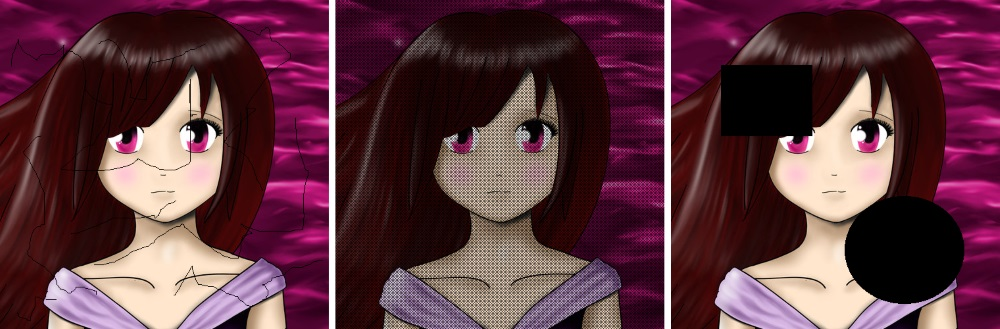OpenCV  3.4.0 Open Source Computer Vision
Inpainting using F-transform

Goal

In this tutorial, you will learn how image inpainting using F-transform works. It consists in:

• basic theory behind,
• three different algorithms.

Introduction

The goal of this tutorial is to show that the inverse F-transform can be used for image reconstruction. By the image reconstruction, we mean a reconstruction of a corrupted image where corruption is everything that the original image does not include. It can be noise, text, scratch, etc. Proposal is to solve the problem of reconstruction with the help of an approximation technique. This means that we will be looking for an approximating image which is close to the given one and at the same time, does not contain what we recognize as the corruption. This task is called image inpainting.

Fuzzy transform application

As I shown in previous tutorial, F-transform is a tool of fuzzy mathematics highly usable in image processing. Let me rewrite the formula using kernel $$g$$ introduced before as well:

$F^0_{kl}=\frac{\sum_{x=0}^{2h+1}\sum_{y=0}^{2h+1} \iota_{kl}(x,y) g(x,y)}{\sum_{x=0}^{2h+1}\sum_{y=0}^{2h+1} g(x,y)},$

where $$\iota_{kl} \subset I$$ centered to pixel $$(k \cdot h,l \cdot h)$$ and $$g$$ is a kernel. For purpose of image processing, a binary mask $$S$$ is used such as

$g^s_{kl} = g \circ s_{kl}$

where $$s_{k,l} \subset S$$. Subarea $$s$$ of mask $$S$$ corresponds with subarea $$\iota$$ of image $$I$$. Operator $$\circ$$ is element-wise matrix multiplication (Hadamard product). Formula is updated to

$F^0_{kl}=\frac{\sum_{x=0}^{2h+1}\sum_{y=0}^{2h+1} \iota_{kl}(x,y) g^s(x,y)}{\sum_{x=0}^{2h+1}\sum_{y=0}^{2h+1} g^s(x,y)}.$

More details can be found in related papers.

Code

/* Sample - Inpainting
* Target is to apply inpainting using F-transform
* on the image "input.png". The image is damaged
* by various types of corruption:
*
* input1 = image & mask1
* input2 = image & mask2
* input3 = image & mask3
*
* Three algorithms "ft::ONE_STEP", "ft::MULTI_STEP"
* and "ft::ITERATIVE" are demonstrated on the
* appropriate type of damage.
*
* ft::ONE_STEP
*
* ft::MULTI_STEP
*
* ft::ITERATIVE
*
* Linear kernel with radius 2 is used for all
* samples.
*/
#include "opencv2/core.hpp"
using namespace std;
using namespace cv;
int main(void)
{
// Input image
// Apply the damage
Mat input1, input2, input3;
// Inpaint with various algorithm
Mat output1, output2, output3, output4;
ft::inpaint(input1, mask1, output1, 2, ft::LINEAR, ft::ONE_STEP);
ft::inpaint(input2, mask2, output2, 2, ft::LINEAR, ft::MULTI_STEP);
ft::inpaint(input3, mask3, output3, 2, ft::LINEAR, ft::MULTI_STEP);
ft::inpaint(input3, mask3, output4, 2, ft::LINEAR, ft::ITERATIVE);
// Save output
imwrite("output1_inpaint.png", output1);
imwrite("output2_inpaint.png", output2);
imwrite("output3_inpaint.png", output3);
imwrite("output4_inpaint.png", output4);
// Save damaged input for comparison
imwrite("input1.png", input1);
imwrite("input2.png", input2);
imwrite("input3.png", input3);
return 0;
}

Explanation

The sample below demonstrates the usage of image inpainting. Three artificial images are created using the same input and three different type of corruption. In the real life usage, the input image will be already presented but here we created it by ourselves.

First of all, we must load our image and three masks used for artificial damage creation.

// Input image

See that mask must be loaded as IMREAD_GRAYSCALE.

In the next step, the masks are used for damaging our input image.

// Apply the damage
Mat input1, input2, input3;

Using the masks, we applied three different kind of corruption on the same input image. Here is the result.input1, input2 and input3

Do not forget that in real life usage, images input1, input2 and input3 are created naturaly and used as the input directly.

Declaration of output images follows. In the following lines, the method of inpainting is applied. Let me explain three different algorithms one by one.

First of them is ONE_STEP.

ft::inpaint(input1, mask1, output1, 2, ft::LINEAR, ft::ONE_STEP);

The ONE_STEP algorithm simply compute direct F-transform ignoring damaged parts using kernel with radius 2 (as specified in the method calling). Inverse F-transform fill up the missing area using values from the components nearby. It is up to you to choose radius which is big enough.

Second is MULTI_STEP.

ft::inpaint(input2, mask2, output2, 2, ft::LINEAR, ft::MULTI_STEP);
ft::inpaint(input3, mask3, output3, 2, ft::LINEAR, ft::MULTI_STEP);

MULTI_STEP algorithm works in the same way but defined radius (2 in this case) is automatically increased if it is found insufficient. If you want to fill up the hole and you are not sure how big radius you need, you can choose MULTI_STEP and let the computer decide. The lowest possible will be found.

Last one is ITERATIVE.

ft::inpaint(input3, mask3, output4, 2, ft::LINEAR, ft::ITERATIVE);

Best choice in majority of cases is ITERATIVE. This way of processing use small radius of basic functions for small kind of damage and higher ones for bigger holes.output1 (ONE_STEP), output2 (MULTI_STEP), output3 (MULTI_STEP), output4 (ITERATIVE)